“The first duty in life is to be as artificial as possible. What the second duty is no one has as yet discovered.” Oscar Wilde# Multivariate Time Series Forcasting with Neural Networks (2) – univariate signal noise mixtures

In this follow up post we apply the same methods we developed previously to a different dataset.

Again, as previously, we make use of the out github hosted package timeseries_utils, and a script:

## Data

The main difference to the previous post is the data. In this post the data is again created artificially. However here, we use a collection of univariate series, which makes this setting particular suitable for arima. The univariate series are composed of composed of a random innovator part and a predictable part, with offsets.

In order for our skill score to be able to cope with the offsets, we need to use the a moving average model as the reference viz. null hypothesis. In this post we use a averaging window of one, as implied by movingAverage=1, which is just the actual series’ previous value.

Results

## Arima

As mentioned, the  defineFitPredict needs to be defined for each forecasting technique. In the case of arima we use defineFitPredict_ARIMA, which is  supplied by our package timeseries_utils.

Now we run the script shown above.

The configuration is this:

The result is: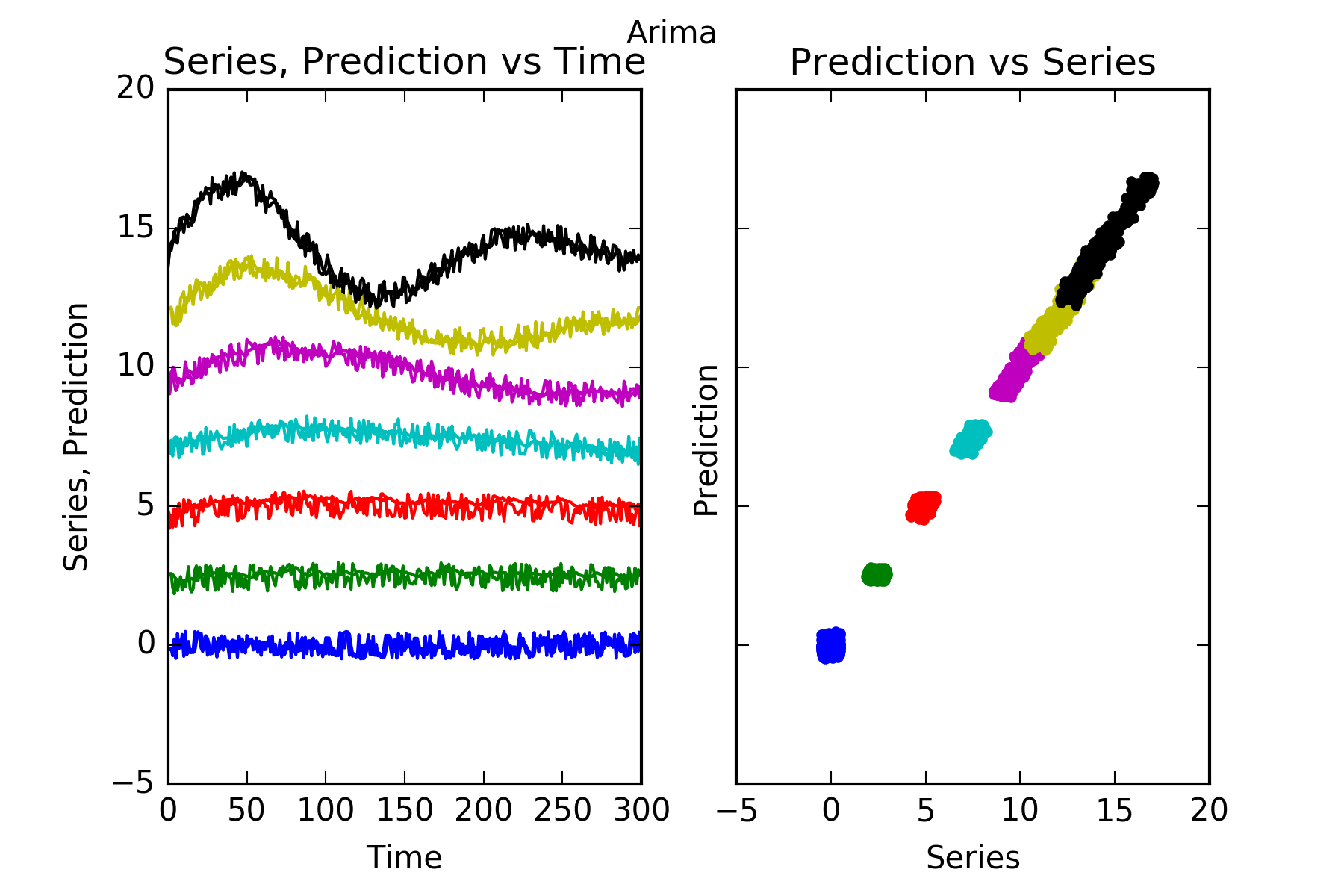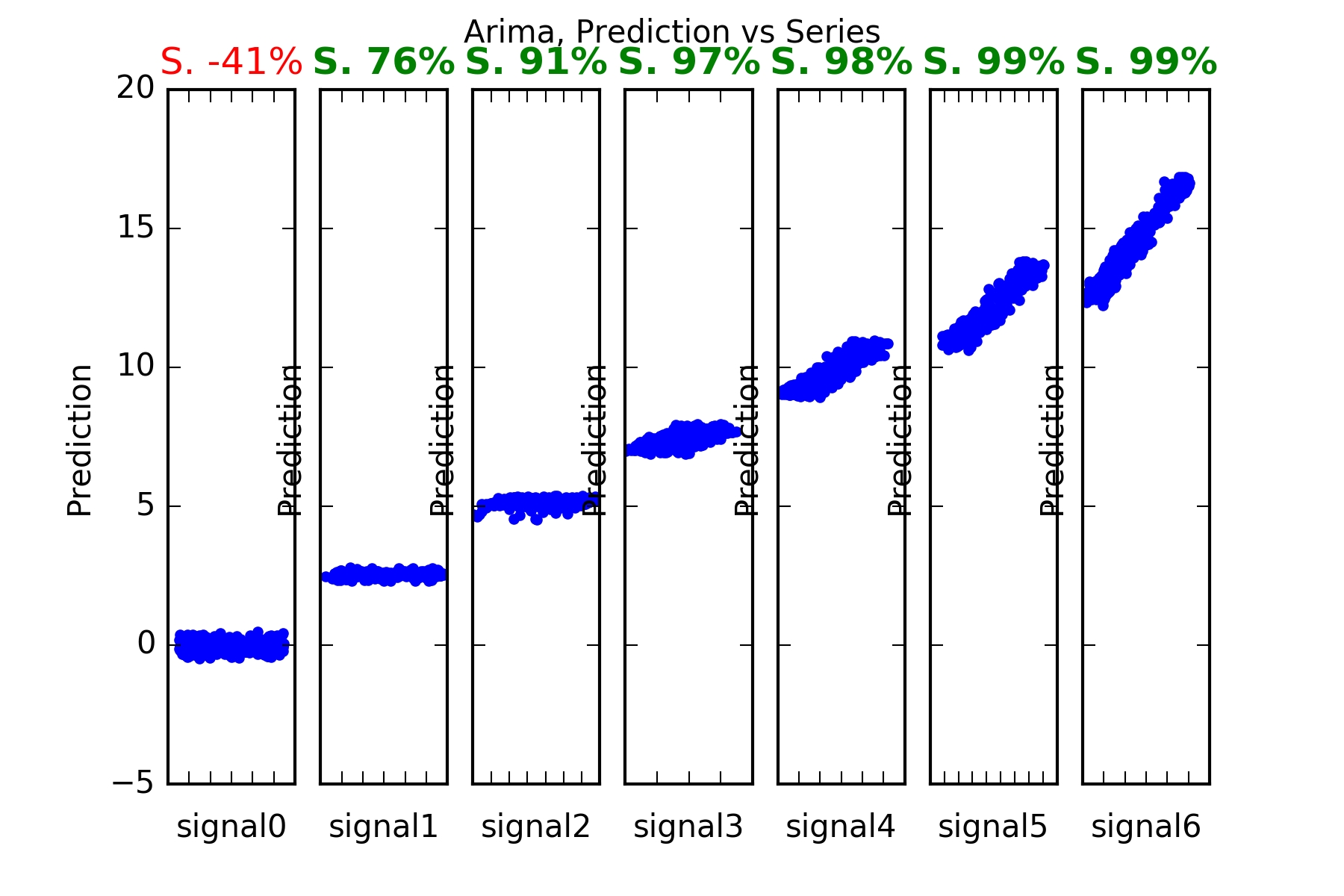Interpretation : As noted above, Arima performs well in this setting, ie with data being a collection of univariate series. It predicts those series with predictable parts, ie series1 to series6. And it cannot predict signal0, which is a random noise innovator.

This is evidenced in the figures, and also in the skill scores(S. in the figures). An skill score of 100% means perfect prediction, 0% skill score means the prediction is as good the alternative reference hypothesis, for which here we have chosen the series’ lagged previous value.

## Dense

As mentioned, the  defineFitPredict needs to be defined for each forecasting technique. In the case of our dense network we usedefineFitPredict_DENSE, which is also supplied by our package timeseries_utils.

Now we run the script shown above.

Note that we’ve turned on differencing, as is evident in the configuration with differencingOrder:1. This improves performance.

The model summary is this:

The result is: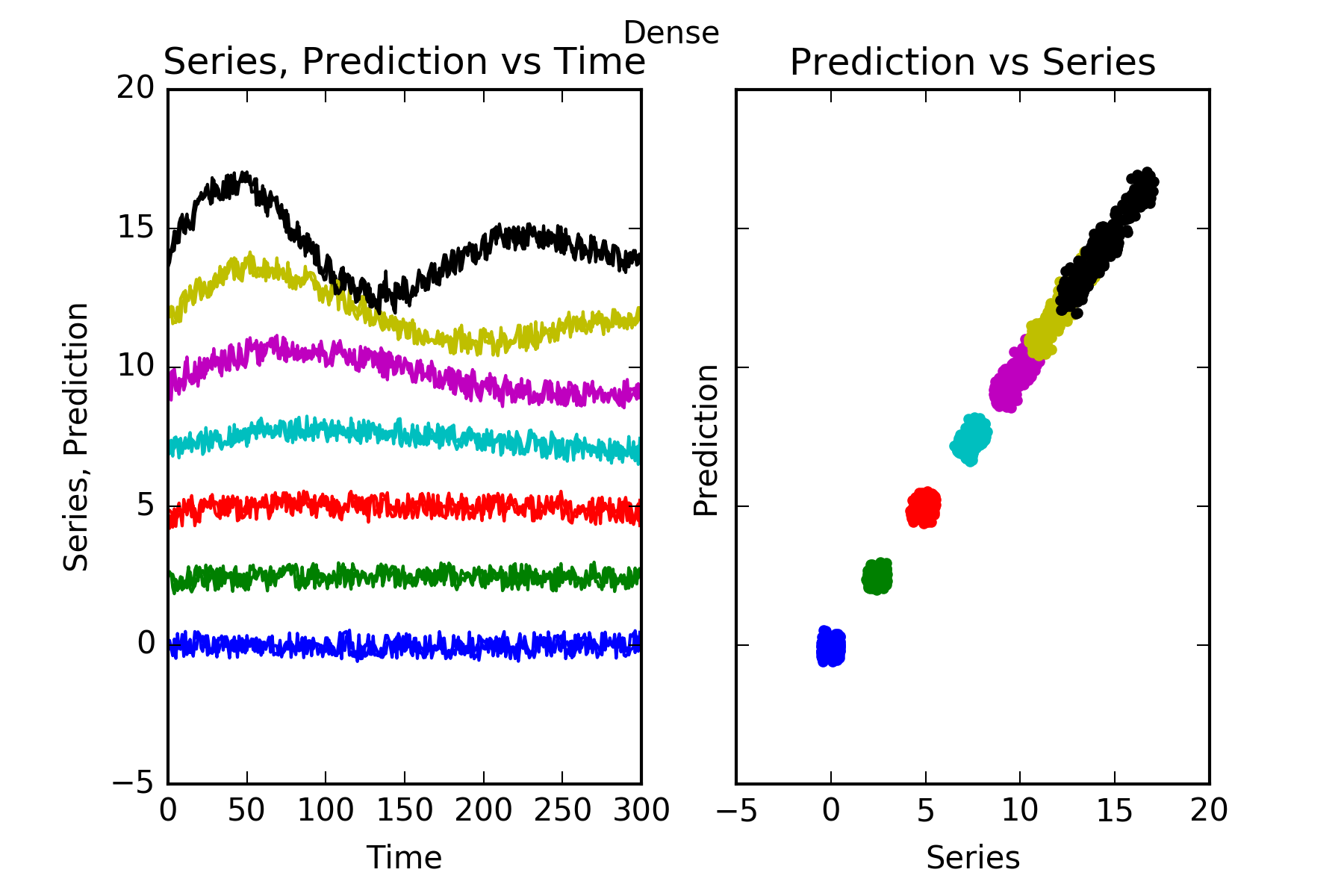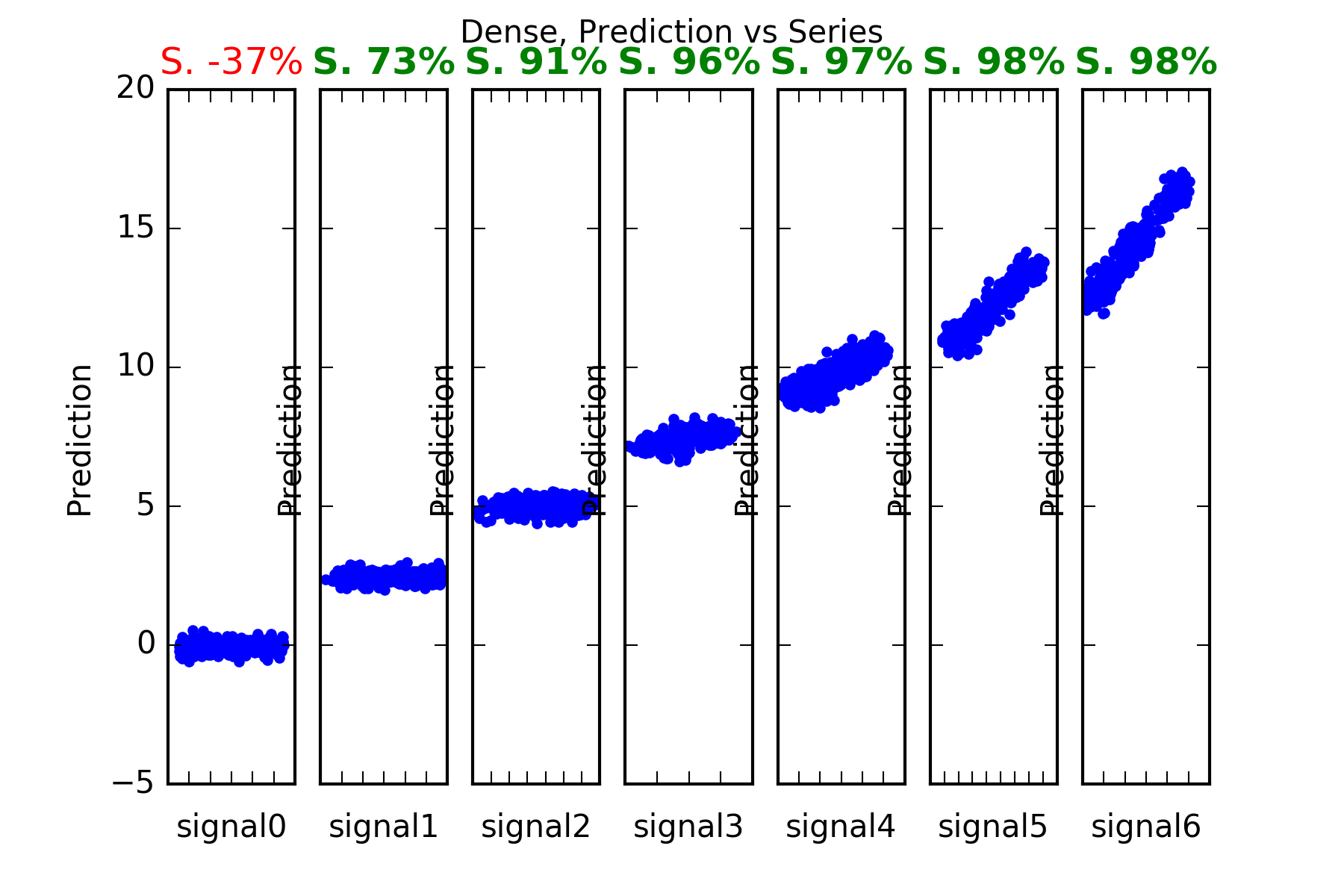Interpretation :  The performance of the Dense prediction is on par with Arima’s.

Its prediction beats the benchmark for those series with predictable parts, ie series1 to series6. And it certainly cannot predict signal0, which is a random noise innovator.

This is evidenced in the figures, and also in the skill scores(Acc. in the figures). An skill score of 100% means perfect prediction, 0% skill score means the prediction is as good as random chance. As stated the only series where the skill is close to 0,  is series signal0.

## LSTM

The model summary is this: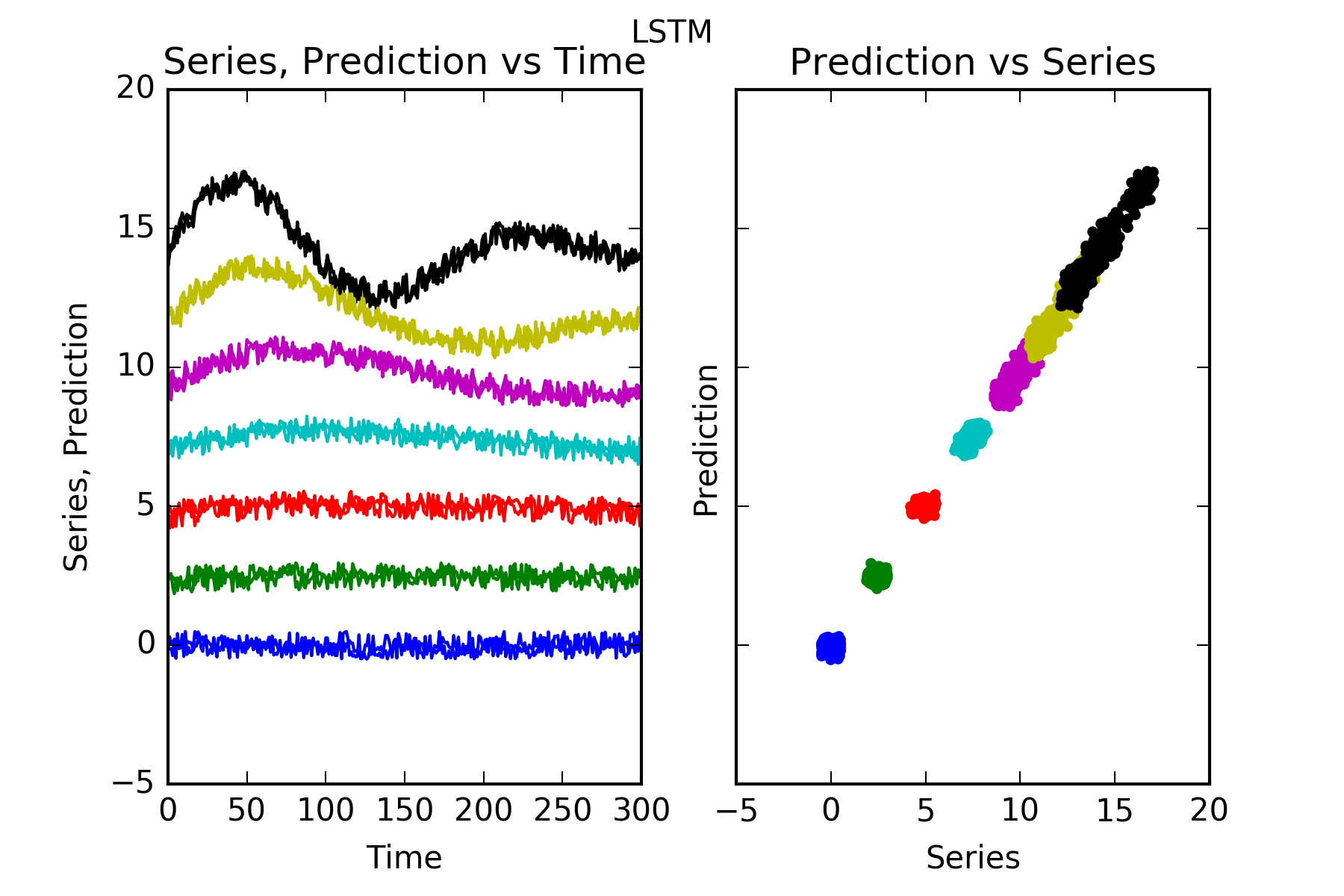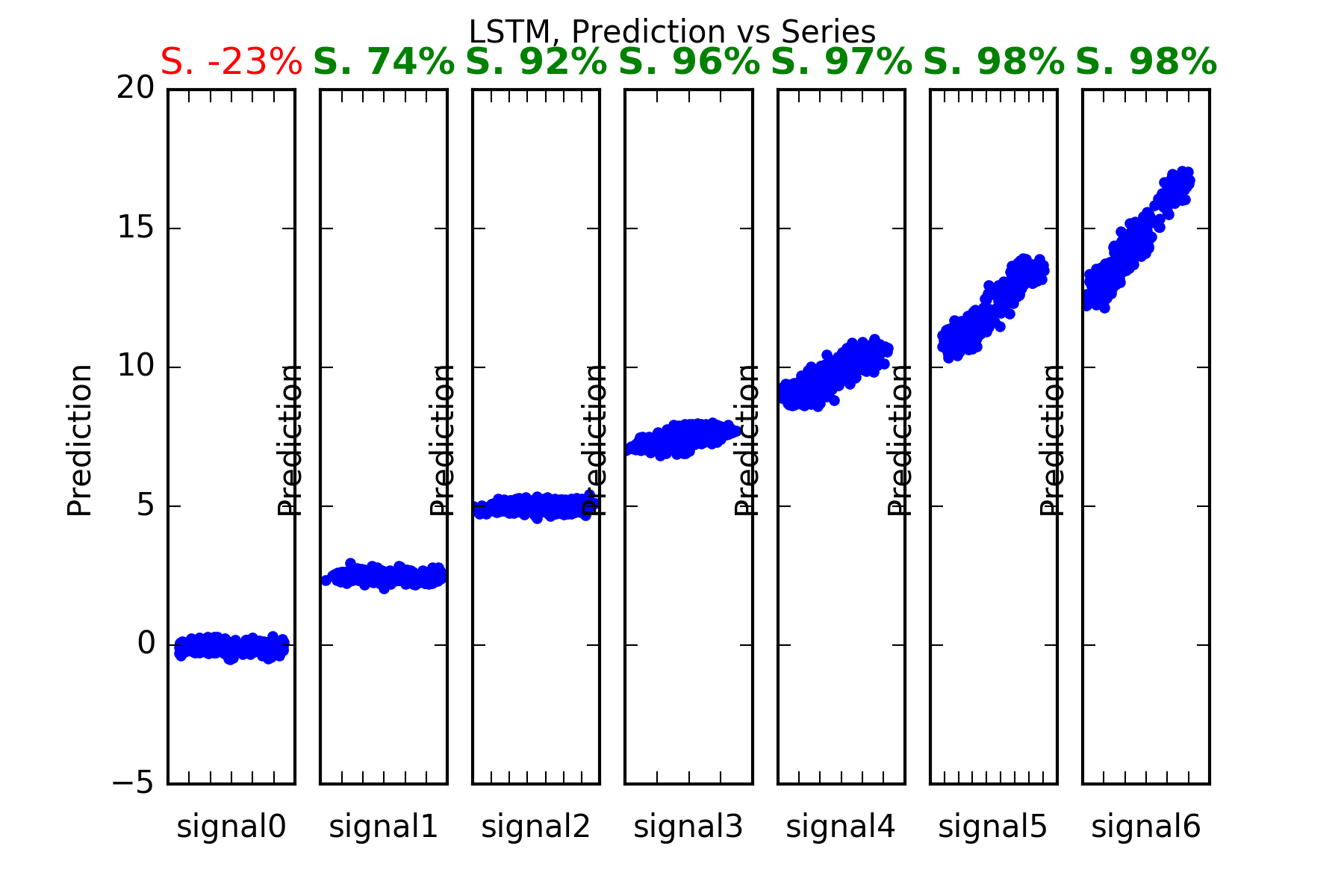Interpretation : Except for the nearly unpredictable signal0, the performance of the Dense prediction is on par with Arima’s and Dense’s.

Its prediction beats the benchmark for those series with predictable parts, ie series1 to series6. And it certainly cannot predict signal0, which is a random noise innovator.

This is evidenced in the figures, and also in the skill scores(S. in the figures). A skill score of 100% means perfect prediction, 0% skill score means the prediction is as good as random chance. As stated the only series where the sklill is close to 0,  is series signal0.

Result summary:

Here’s the summary table: